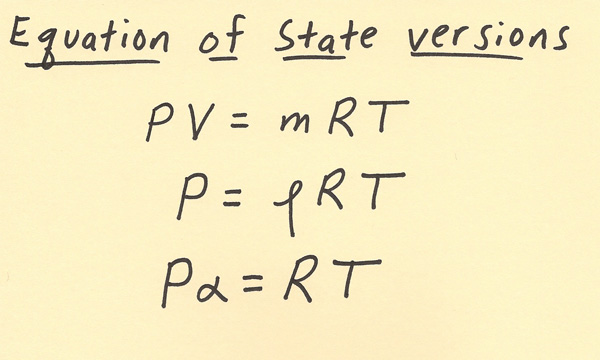[--MAIN HOME--] [--ALL HABYHINTS--] [--FACEBOOK PAGE--]

 HABYTIME MINI LECTURE 71: EQUATION OF STATE

METEOROLOGIST JEFF HABY

The equation of state is used for gases to compare various properties that they have including pressure, volume, mass, temperature, density, and specific volume. Each of these is defined below:

Pressure (P): force exerted by air per unit area (units can be expressed in Newtons/meter^2)

Volume (V): amount of space occupied by a gas (units can be expressed in meter^3 or cm^3)

Mass (m): amount of gas present (units can be expressed in kilograms or grams)

Temperature (T): a measured amount of heat present (units are commonly expressed in Celsius or Kelvins)

Density: the amount of gas per volume gas is within (units can be expressed in kilograms/meter^3 or grams/cm^3)

Specific Volume: the volume gas is in per amount of gas (units can be expressed in meters^3/kilogram or cm^3/grams). Density and specific volume are inverses of each other.

There is also a gas constant R that balances the relationship among the variables.

The equation of state is an ideal equation meaning there is an assumption that the gases perfectly obey the gas laws. Thus, the equation of state is also called the ideal gas law. Gases that obey the equation of state ideally have the following relationship between variables in the equations below. The equations below are three common arrangements of the equation of state.

The top equation has Pressure times Volume equals mass times a gas constant (R) times temperature

The middle equation has Pressure equals density times a gas constant (R) times temperature

The bottom equation has Pressure times specific volume equals a gas constant (R) times temperature

All three rewritings are variations of the same equation. They are rewritten using various forms of the density equation. Density = mass / Volume. The top equation having both sides divided by Pressure results in only Pressure being left on the left side of the equation and density showing on the right side of the equation in the middle equation. In the bottom equation, the specific volume results from taking the top equation and dividing both sides by the mass.

The equation of state basically relates pressure and density and temperature. If one of these variables changes, then the others will typically change also. Below are some common relationships that typically occur, and can vary depending on if air is trapped in a closed container or if it is allowed to expand or contract:

Temperature increase: often results in Pressure increase, Volume increase, Density decrease

It helps to think of a hot air balloon filling with hot air for the above relationships

Temperature decrease: often results in Pressure decrease, Volume decrease, Density increase

Pressure increase: often results in temperature increase if volume is fixed, and a volume increase if volume can expand

Pressure decrease: often results in temperature decrease if volume is fixed, and a volume decrease if volume can contract

Density decrease: often results in a temperature increase if volume is fixed in order to maintain the same pressure

Density increase: often results in a temperature decrease if volume is fixed in order to maintain the same pressure

The equation of state can work differently in the atmosphere than in a laboratory setting since in the atmosphere typically variables can not be held constant. For example, volume can be held constant in the lab while in the atmosphere the air will be free to expand or contract. In the lab setting, the advantage to holding a variable constant, is that the direct or indirect relationship between the other variables in the equation of state can be studied. This results in the following relationships using the (PV=mRT) equation:

Volume held constant: when pressure increases, temperature increases and when pressure decreases, temperature decreases. This is known as a direct relationship since when one goes up the other also goes up and when one goes down the other also goes down

Pressure held constant: when volume increase, temperature increases and when volume decreases, temperature decreases. This is also a direct relationship

Temperature held constant: when pressure increases, volume decreases and when pressure decreases, volume increases. This is an indirect relationship since when one goes up and other goes down.

Understanding these relationships can help understand the more complication relationships among the variables that occur in the atmosphere.